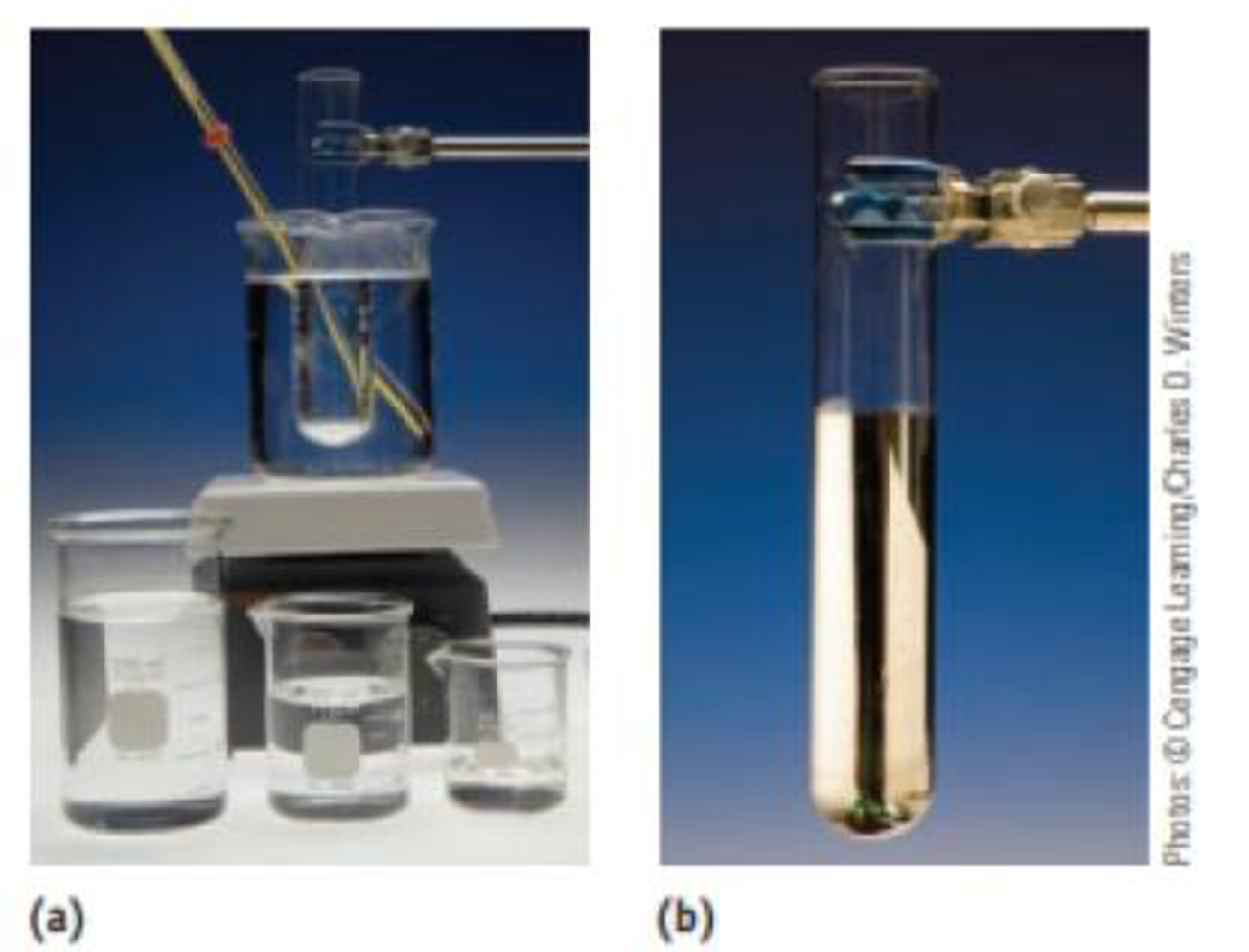Chapter 3, Problem 93IL

Chapter
Section
Textbook Problem

The Tolien’s test for the presence of reducing sugars (say, in a urine sample) involves treating the sample with silver ions in aqueous ammonia. The result is the formation of a silver mirror within the reaction vessel if a reducing sugar is present. Using glucose, C6H12O6, to illustrate this test, the oxidation-reduction reaction occurring isC6H12O6 (aq) + 2 Ag+(aq) + 2OH–(aq) → C6H12O7(aq) + 2 Ag(s) + H2O(ℓ) What has been oxidized, and what has been reduced? What is the oxidizing agent, and what is the reducing agent?Tolien's test. The reaction of silver ions with a sugar such as glucose produces metallic silver. (a) The set-up for the reaction. (b) The silvered test tube

Interpretation Introduction

Interpretation:

• Oxidized and reduced compound has to be identified in the given reaction.
• Oxidizing and reducing agent should be found.

Concept introduction:

An oxidizing agent gains the electrons and is reduced in a chemical reaction and it is electron acceptor.

A reducing agent loses electrons and is oxidized in a chemical reaction and it is electron donor.

The oxidation state is called as oxidation number, which describes degree of oxidation (loss of electrons) of an atom in a chemical compound.  Theoretically, the oxidation state is positive, negative or zero.

Explanation

The given reaction is shown below,

Ag+ is reduced to Ag, because it gets the electron, the oxi

Still sussing out bartleby?

Check out a sample textbook solution.

See a sample solution

The Solution to Your Study Problems

Bartleby provides explanations to thousands of textbook problems written by our experts, many with advanced degrees!

Get Started

Find more solutions based on key concepts